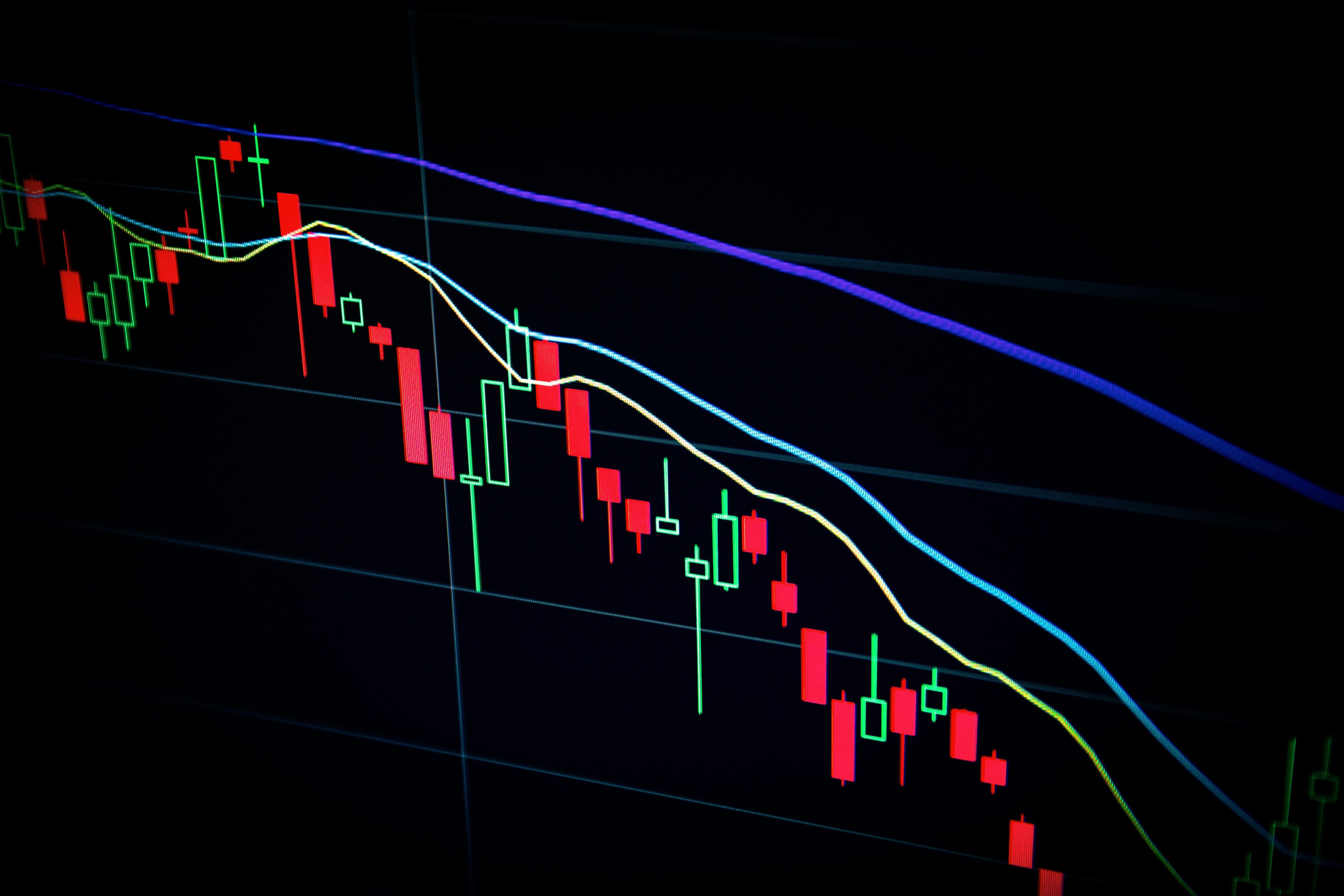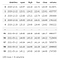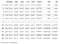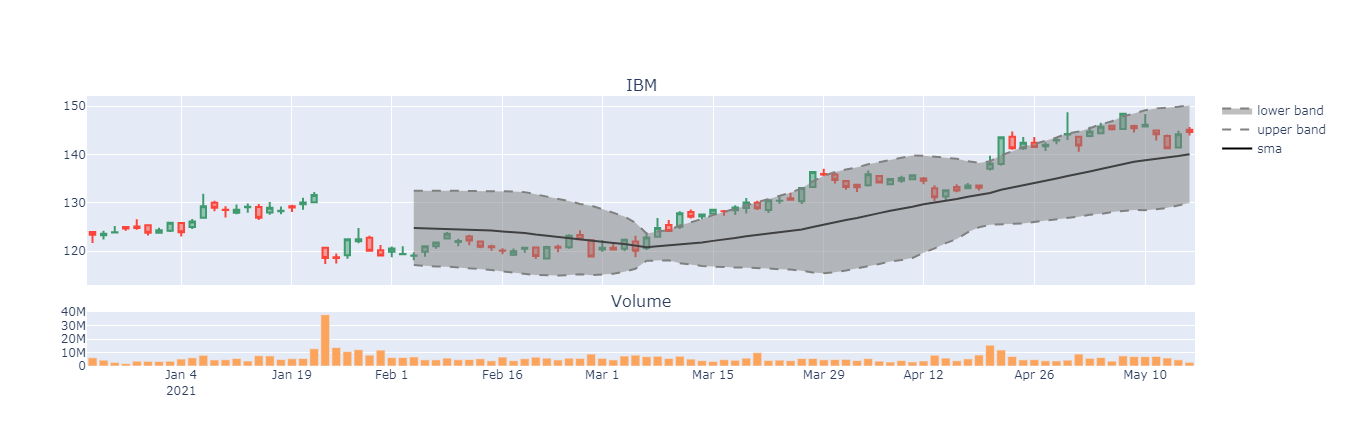# Plotting Bollinger Bands with Plotly Graph ObjectsPhoto by Maxim Hopman on Unsplash

For this blog, I will demonstrate how to plot Bollinger Bands using Plotly. Bollinger bands contain upper/lower bounds(±2 standard deviations) from the moving average of stock data. I will break down this tutorial blog into three steps:

1. Obtain Data
Use AlphaVantage for IBM historical data
2. Calculate Moving Averages and Standard Deviation
Calculate the Moving Average, Upper Band, and Lower Band
3. Plot with Plotly
Add layers on top of the candlestick plot

For the simplicity of this blog, I will use the demo version of the AlphaVantage API. AlphaVantage offers free API keys for stock data, and set-up is super easy. The demo API call will retrieve 100 data points(100 days from the present) for the IBM stock.

`import requestsimport jsonimport numpy as npimport pandas as pd# AlphaVantage Demo IBM APIurl = 'https://www.alphavantage.co/query?function=TIME_SERIES_DAILY&symbol=IBM&apikey=demo'response = requests.get(url)# Load text to clean json objectjson_load = json.loads(response.text)# 'Time Series (Daily)' consists the time stamps for the demodf = pd.DataFrame(json_load['Time Series (Daily)']).T# Rename column names & change datetime to pandas datetimedf.reset_index(inplace=True)df.columns = ['datetime', 'open', 'high', 'low', 'close', 'volume']df['datetime'] = pd.to_datetime(df['datetime'])display(df)`display(df);

Bollinger Bands include a moving average with upper and lower bounds(±2 standard deviations) away from the running average. The moving average can be calculated using the Pandas helper function rolling with a set WINDOW size. For this blog, I will set WINDOW to 30.

It’s important to note, Bollinger Bands use the population method when calculating standard deviations, and therefore the denominator for the sigma calculation is n and not n -(minus) 1. When using the Pandas helper function, rolling with std, set degrees of freedom(ddof) to 0.

`WINDOW = 30df['sma'] = df['close'].rolling(WINDOW).mean()df['std'] = df['close'].rolling(WINDOW).std(ddof = 0)display(df)`display(df);

With a window of 30, the first 29 observations for the moving average and standard deviations should be NULL due to the lack of data points prior to the beginning data points.

After feature engineering the moving averages and standard deviation, I have all I need to plot the Bollinger Bands. The Bollinger Bands consist of the moving average(center), with the upper and lower bounds being ± 2 standard deviations from the running average.

Plotting with plotly, matplotlib, folium, or other python visualization packages adds layers on a canvas. The code block below may seem overwhelming, but you can view it as a sequential canvas painting. First, create two canvases, one for candlesticks and one for volume. And for each canvas, build plots on top of each other.fig.show();

Hello! My name is Albert Um.

## More from Albert Um

Hello! My name is Albert Um.